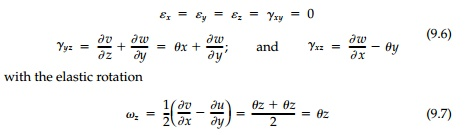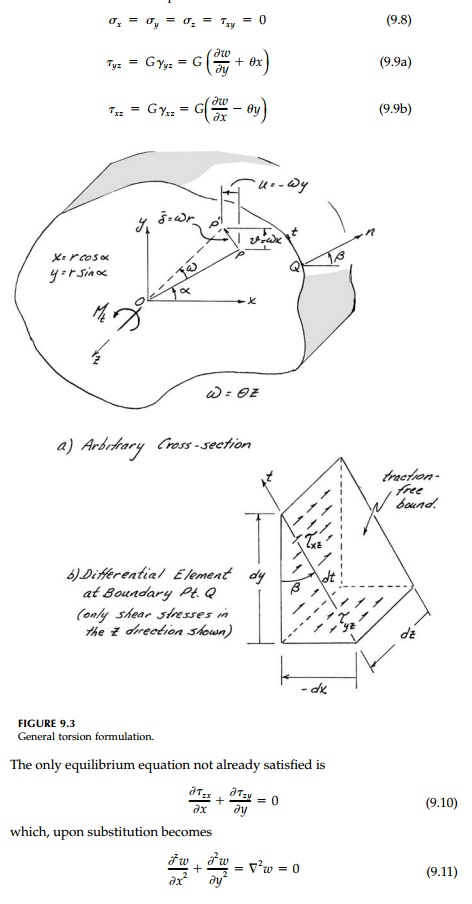Home | | Mechanics of Solids | St. VenantŌĆÖs Formulation (Noncircular Cross Sections)

# St. VenantŌĆÖs Formulation (Noncircular Cross Sections)

Torsion: Elementary (Linear) Solution : The general problem of a shaft under torque is obviously of great practical importance.Torsion

Elementary (Linear) Solution

The general problem of a shaft under torque is obviously of great practical importance. The linear solution, as presented in a first course in solid mechanics, assumes that for a circular cross-section, radical lines remain straight when the torque is applied. Thus, as shown in Figure 9.1, two neighboring cross-sections, dz apart rotate relative to each other by a small angle of twist dŽē. Thus, longitudinal straight lines become helixes at the angle of shear strain, ╬│, related to the angle of twist by the circumferential movement:St. VenantŌĆÖs Formulation (Noncircular Cross Sections)

The approach taken by St. Venant to the torsion problem provides a neat demonstration of the semi-inverse method of solving the elasticity equations for the displacement vector field. Although results in closed form can only be found for a few simple shapes, the St. Venant formulation, because it deals with displacements, is intuitive and gives physical insight into the complica-tions introduced by nonconstant axial displacements required to satisfy the boundary conditions for noncircular cross-sections.**

That longitudinal cross-sections, if not circular, deform or ŌĆ£warpŌĆØ is shown in Figure 9.2. Assuming the rod is cylindical in the z direction (and not tapered) and that projections of the cross-section on the xy plane rotate rigidly at a con-stant rate of twist, ╬Ė, St. Venant, pioneering the inverse method, postulated the displacement field (shown in Figure 9.3):as the solution (the answer). Thus the warp of the cross-section, w, is left as an unknown function (i.e., the warping function) to be adjusted to satisfy the boundary condition dictated by the shape. In terms of strains, he therefore postulated that:being the total angle of twist of the cross-section. Geometric compatibility is automatically satisfied because this formulation assumes continuous and smooth displacements.

From the constitutive relationships:Thus any harmonic function for the longitudinal displacement or warping is a possible elastic field solution for the general torsion problem if we can find the shape of the cross-section where the traction-free boundary condition is satisfied.

The resultant twisting moment on the ends of the rod is:is often called the ŌĆ£torsional constantŌĆØ and GJ expresses the ŌĆ£torsional rigidityŌĆØ of the bar.

Considering an arbitrary cross-section such as given in Figure 9.3a, there can be no shear Žänt at the surface. Thus considering an element at any bound-ary point Q, longitudinal equilibrium requires that:where dx is negative in Figure 9.3b since a positive dt causes x to decrease. Therefore, the boundary condition becomesSolutions by St. Venant

The St. Venant formulation therefore leads to the search for harmonic functions w(x,y) that satisfy Equation (9.16). In general this Neumann boundary condi-tion is difficult to apply especially for nonsymmetric cross-sections where the center of rotation is not obvious. This illustrates the inherent difficulty, referred to in Previews, in employing the displacement strategy for elasticity solu-tions where the boundary conditions involve stresses.

Nevertheless, a few simple harmonic functions for the warping are man-ageable leading to practical inverse solutions in closed form. The most obvi-ous is to assume the simplest solution to the Laplace equation:and we have a circular shaft and the elementary linear solution already dis-cussed for plane sections is correct. From Equation (9.13), J = Ip = ŽĆ r4  / 2 and all the standard formulae follow.

b. w = Kxy

The hyperbolic function, w  = Kxy, is harmonic and therefore satisfies the equilibrium requirement of Equation (9.6). The boundary condition [Equation (9.16)] gives: x(K + ╬Ė ) dx  + y (K - ╬Ė ) dy which, when integrated, gives the required boundary shape:which is somewhat counterintuitive but will be obvious from the analogies drawn later.

If the ends of the bar are not restrained from warping, there will be no normal stresses. However, if one or both ends are restrained such as for a built-in shaft, normal stresses will be induced similar to those that would result from equal and opposite bending moments and are, therefore, sometimes called the ŌĆ£bending stresses induced by torsion.ŌĆØ

Study Material, Lecturing Notes, Assignment, Reference, Wiki description explanation, brief detail
Civil : Principles of Solid Mechanics : Torsion : St. VenantŌĆÖs Formulation (Noncircular Cross Sections) |

Related Topics